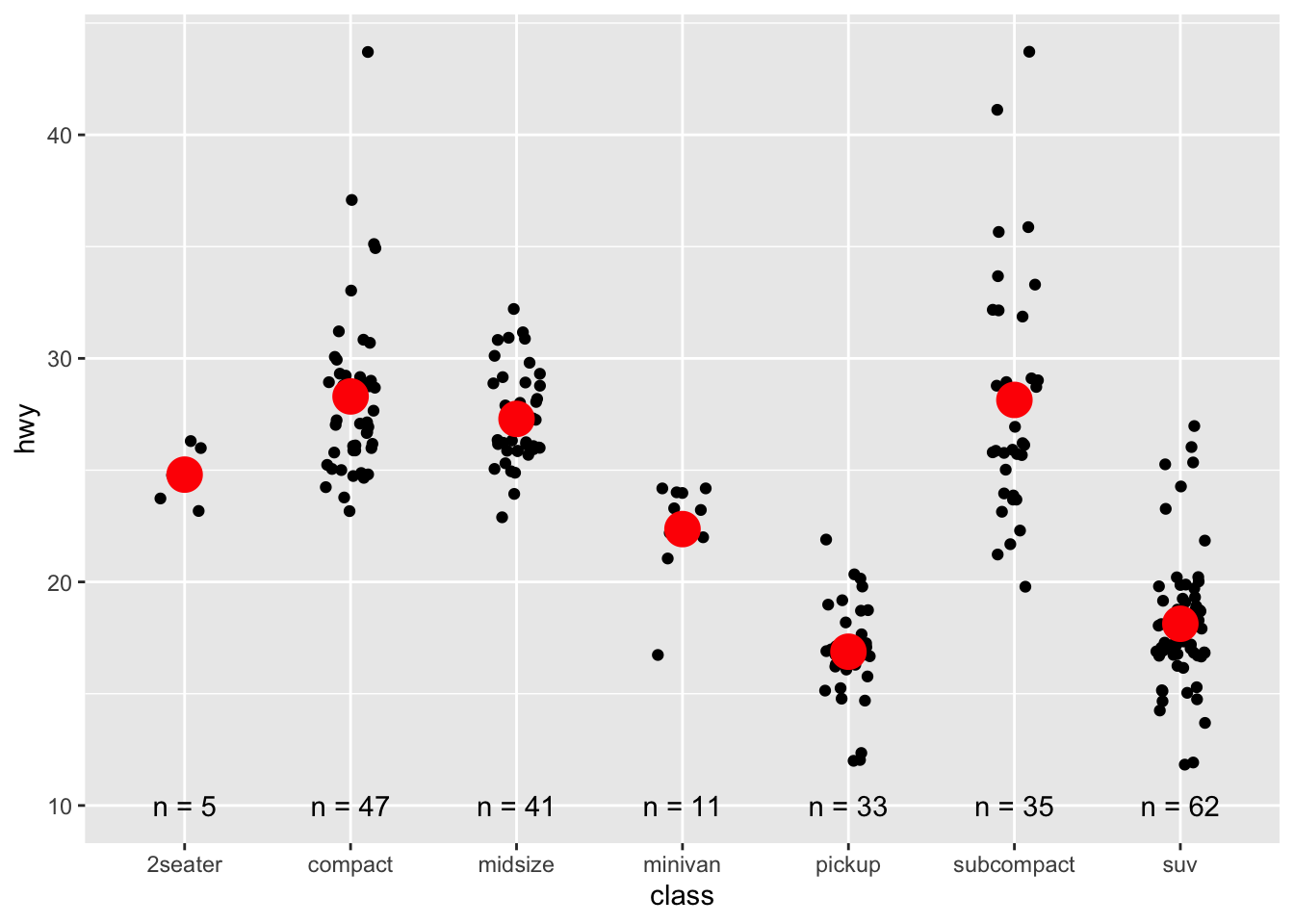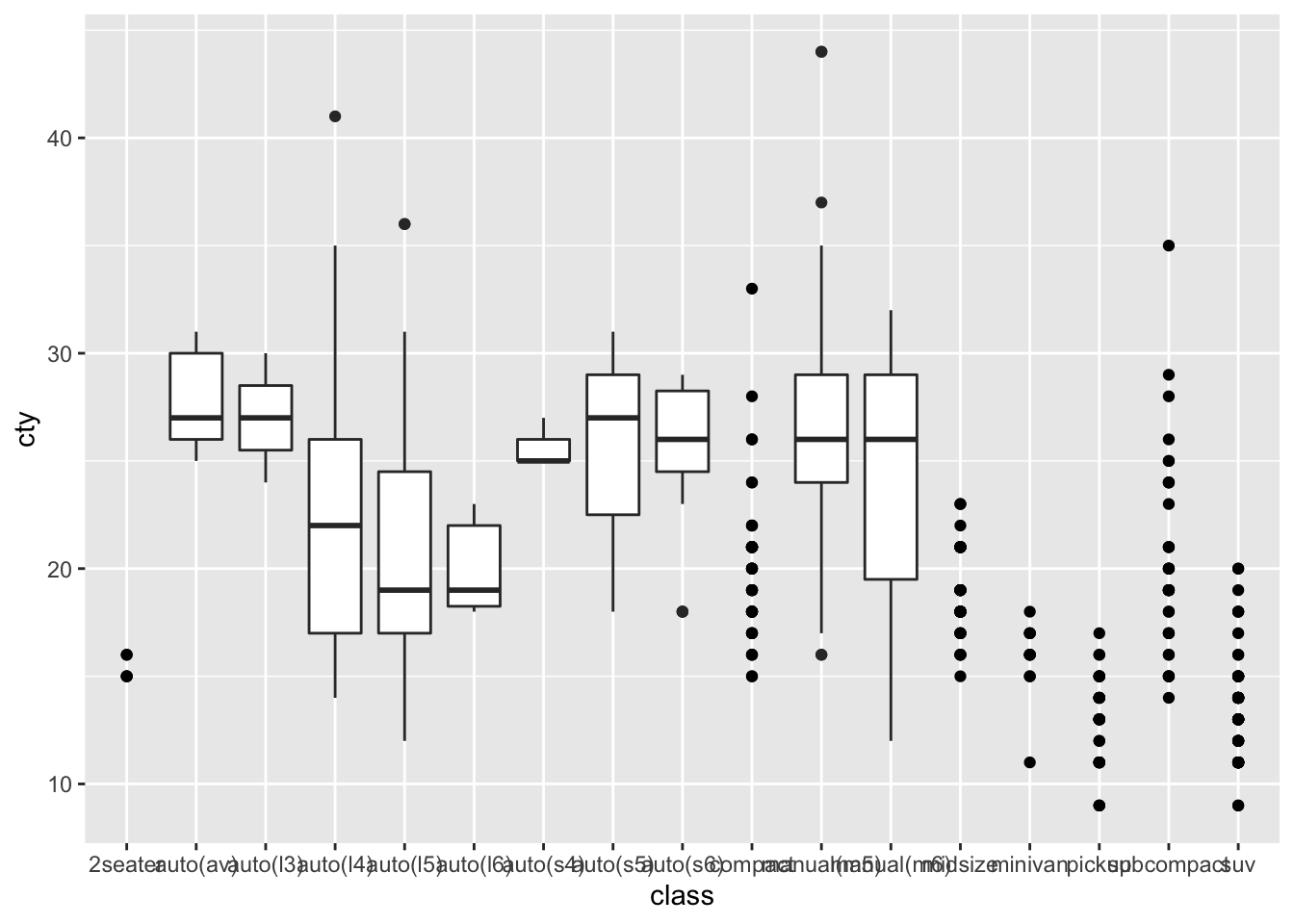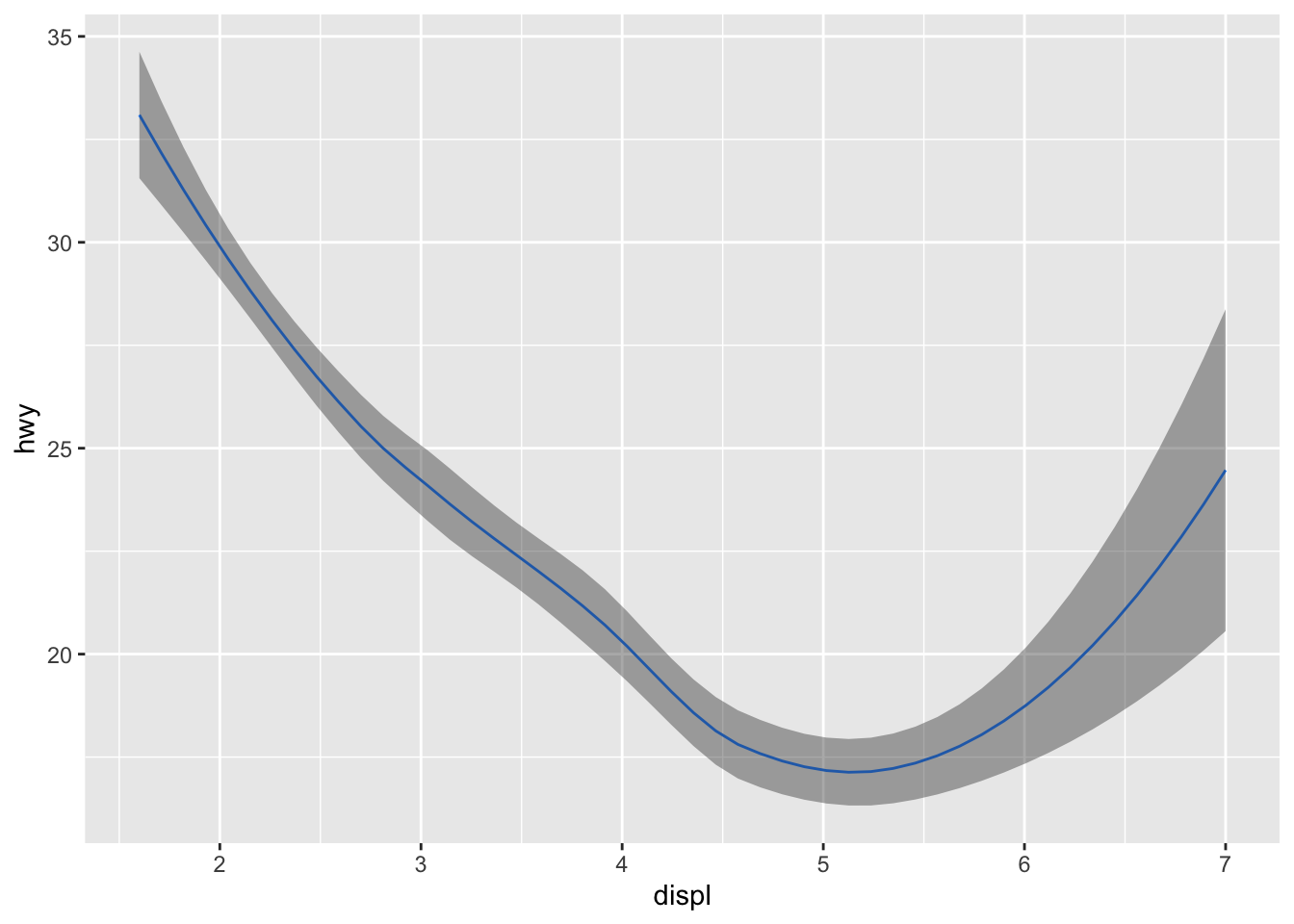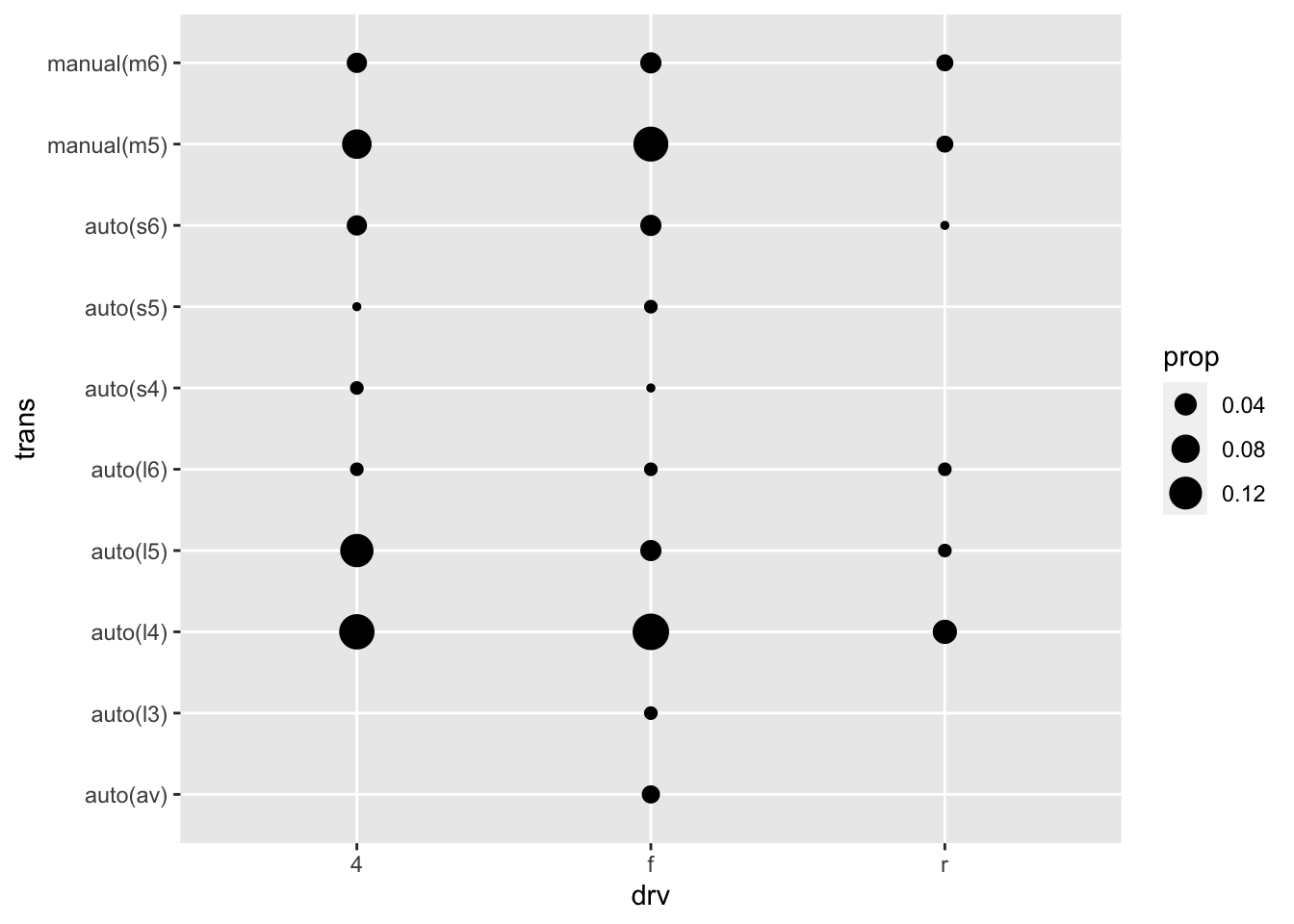# 14 Build a plot layer by layer

## 14.1 Exercises

1. The first two arguments to ggplot are `data` and `mapping`. The first two arguments to all layer functions are `mapping` and `data`. Why does the order of the arguments differ? (Hint: think about what you set most commonly.)

• Commonly, you first set the data in `ggplot()` and then set aesthetics inside your layer functions, like `geom_point()`, `geom_boxplot()`, or `geom_histogram()`.

2.

``````library(dplyr)
class <- mpg %>%
group_by(class) %>%
summarise(n = n(), hwy = mean(hwy))``````
``````mpg %>%
ggplot(aes(class, hwy)) +
geom_jitter(width = 0.15, height = 0.35) +
geom_point(data = class, aes(class, hwy),
color = "red",
size = 6) +
geom_text(data = class, aes(y = 10, x = class, label = paste0("n = ", n)))``````• I plotted 3 different layers: jittered points, red point for the summary measure, mean, and text for the sample size (n).

## 14.2 Exercises

1. Simplify the following plot specifications:

``````####################################
####################################
# ggplot(mpg) +
#   geom_point(aes(mpg\$displ, mpg\$hwy))

# The above can be simplified:
# ggplot(mpg) +
#   geom_point(aes(displ, hwy))
####################################
####################################

####################################
####################################
# ggplot() +
#  geom_point(mapping = aes(y = hwy, x = cty),
#             data = mpg) +
#  geom_smooth(data = mpg,
#              mapping = aes(cty, hwy))

# The above can be simplified:
# ggplot(mpg, aes(cty, hwy)) +
#  geom_point() +
#  geom_smooth()
####################################
####################################

####################################
####################################
# ggplot(diamonds, aes(carat, price)) +
#   geom_point(aes(log(brainwt), log(bodywt)),
#              data = msleep)

# The above can be simplified:
# msleep_processed <- msleep %>%
#   mutate(brainwt_log = log(brainwt),
#          bodywt_log = log(bodywt))

# ggplot(diamonds, aes(carat, price)) +
#   geom_point(aes(brainwt_log, bodywt_log),
#              data = msleep_processed)
####################################
####################################``````

2. What does the following code do? Does it work? Does it make sense? Why/why not?

``````ggplot(mpg) +
geom_point(aes(class, cty)) +
geom_boxplot(aes(trans, hwy))``````• It plots points of `class` vs `cty` and then a boxplot of `trans` vs `hwy`. It doesn’t make sense to plot layers with different `x` and `y` variables.

3. What happens if you try to use a continuous variable on the x axis in one layer, and a categorical variable in another layer? What happens if you do it in the opposite order?

• Not sure

## 14.3 Exercises

1,2,3 omitted.

1. Starting from top left, clockwise direction:
• `geom_violin()`, `geom_point()`, `geom_point()`, `geom_path()`, `geom_area()`, `geom_hex()`.

## 14.4 Exercises

1.

``````mod <- loess(hwy ~ displ, data = mpg)
smoothed <- data.frame(displ = seq(1.6, 7, length = 50))
pred <- predict(mod, newdata = smoothed, se = TRUE)
smoothed\$hwy <- pred\$fit
smoothed\$hwy_lwr <- pred\$fit - 1.96 * pred\$se.fit
smoothed\$hwy_upr <- pred\$fit + 1.96 * pred\$se.fit

smoothed %>%
ggplot(aes(displ, hwy)) +
geom_line(color = "dodgerblue1") +
geom_ribbon(aes(ymin = hwy_lwr,
ymax = hwy_upr),
alpha = 0.4)``````2. From left to right,

`stat_ecdf()`, `stat_qq()`, `stat_function()`

3.

``````mpg %>%
ggplot(aes(drv, trans)) +
geom_count(aes(size = after_stat(prop), group = 1)) ``````## 14.5 Exercises

1. According to the help page, `position_nudge()` is generally useful for adjusting the position of items on discrete scales by a small amount. Nudging is built in to geom_text() because it’s so useful for moving labels a small distance from what they’re labelling.

2. Not sure

3. `geom_jitter()` adds a small amount of random variation to the location of each point. It is useful for looking at all the overplotted points. On the other hand, `geom_count()` counts the number of overlapping observations at each location. It is useful for understanding the number of points in a location.

4. Stacked area plot seems useful when you want to portray an area whereas a line plot seems useful when you just need a line.